Open In App
Related Articles

# Trigonometric Ratios

Trigonometry is all about triangles or to be more precise the relationship between the angles and sides of a right-angled triangle. There are three sides of a triangle named Hypotenuse, Adjacent, and Opposite. The ratio between these sides based on the angle between them is called Trigonometric Ratio.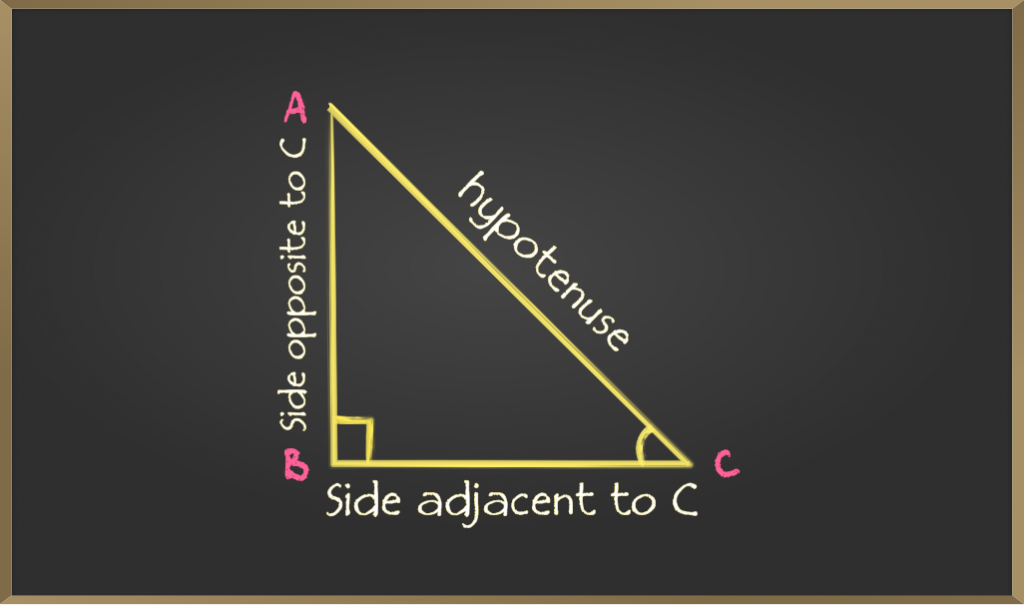As given in the figure in a right-angle triangle

• The side opposite the right angle is called the hypotenuse
• The side opposite to an angle is called the opposite side
• For angle C opposite side is AB
• For angle A opposite side is BC
• The side adjacent to an angle is called the adjacent side
• For angle C adjacent side is BC
• For angle A adjacent side is AB

## What are Trigonometric Ratios?

There are 6 basic trigonometric relations that form the basics of trigonometry. These 6 trigonometric relations are ratios of all the different possible combinations in a right-angled triangle.

These trigonometric ratios are called

• Sine
• Cosine
• Tangent
• Cosecant
• Secant
• Cotangent

The mathematical symbol θ is used to denote the angle.

## Sine (sin)

Sine of an angle is defined by the ratio of lengths of sides which is opposite to the angle and the hypotenuse. It is represented as sin θ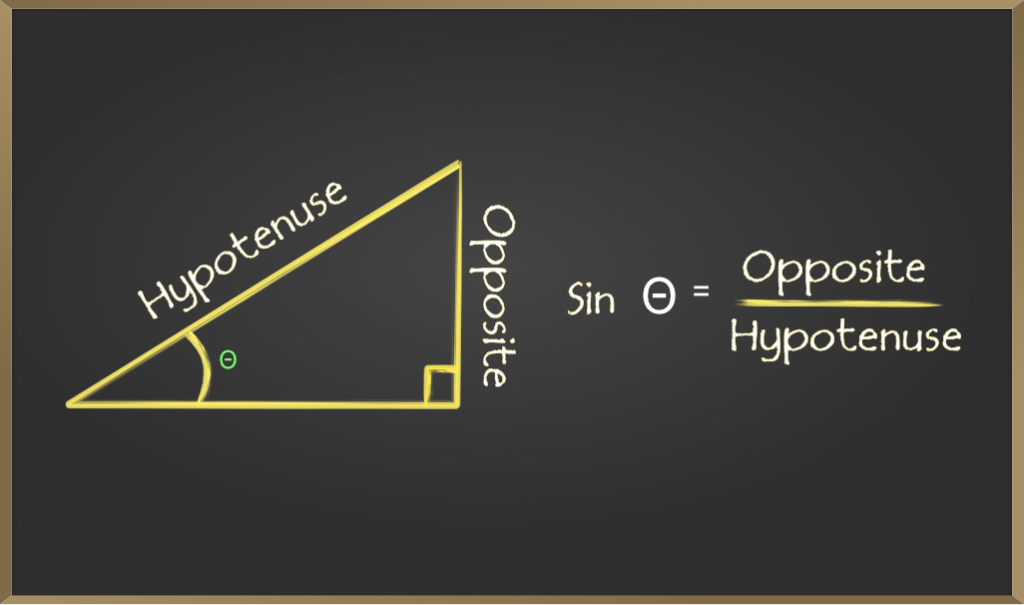## Cosine (cos)

Cosine of an angle is defined by the ratio of lengths of sides which is adjacent to the angle and the hypotenuse. It is represented as cos θ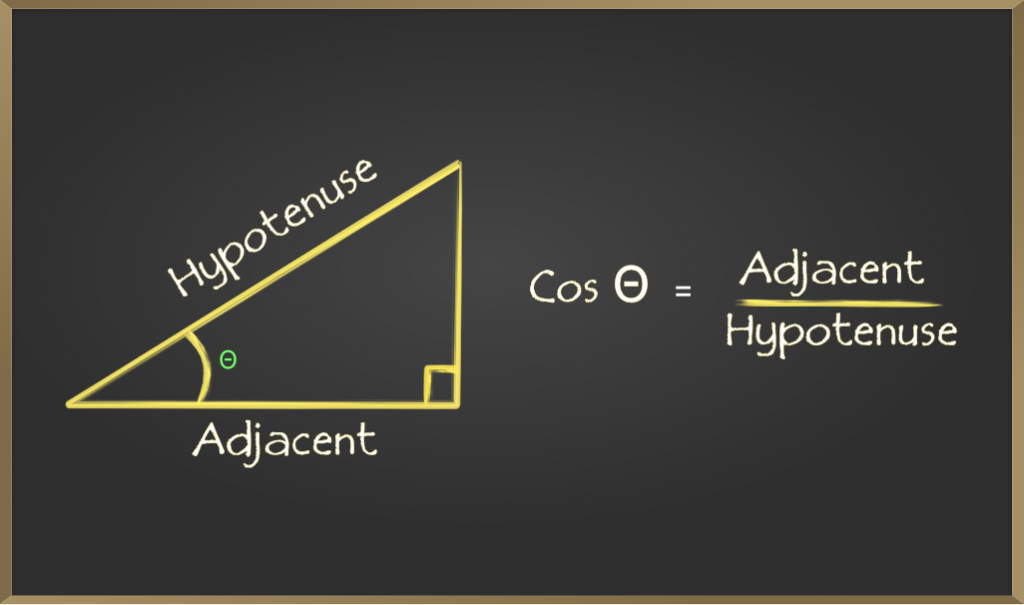## Tangent (tan)

Tangent of an angle is defined by the ratio of the length of sides which is opposite to the angle and the side which is adjacent to the angle. It is represented as tan θ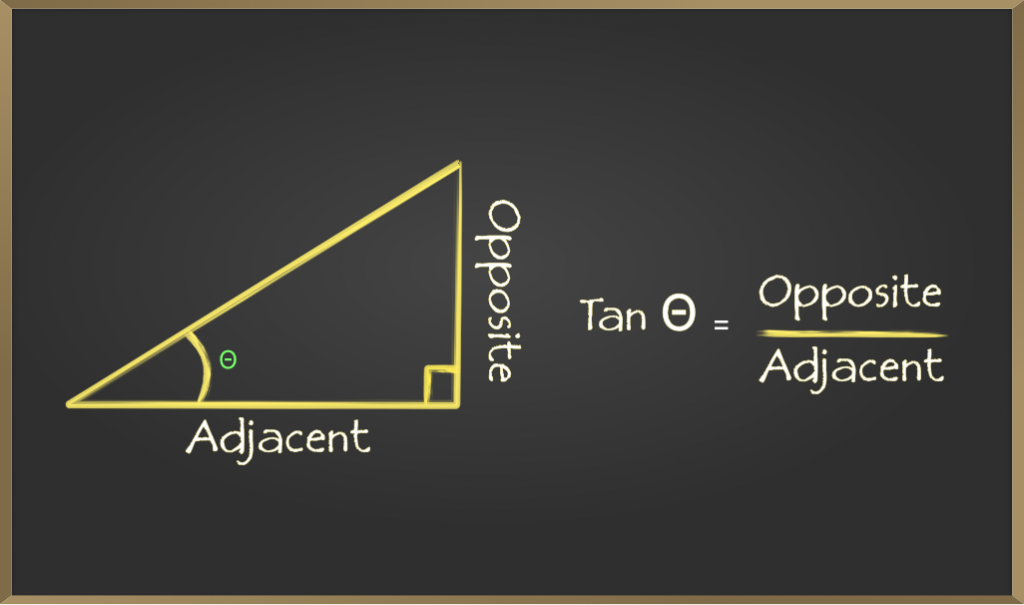## Cosecant (cosec)

Cosecant of an angle is defined by the ratio of the length of the hypotenuse and the side opposite the angle. It is represented as cosec θ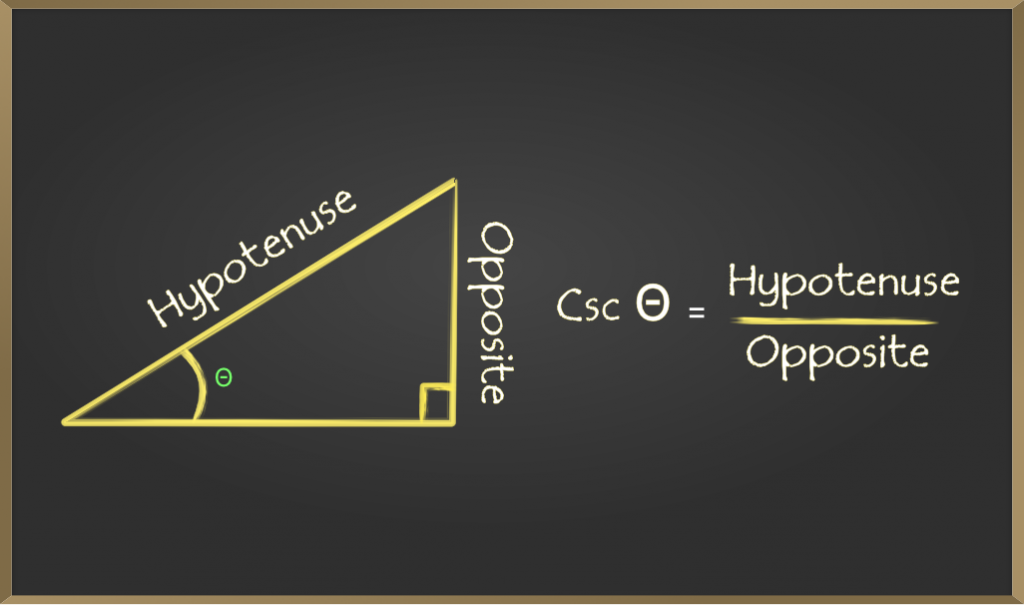## Secant (sec)

Secant of an angle is defined by the ratio of the length of the hypotenuse and the side and the side adjacent to the angle. It is represented as sec θ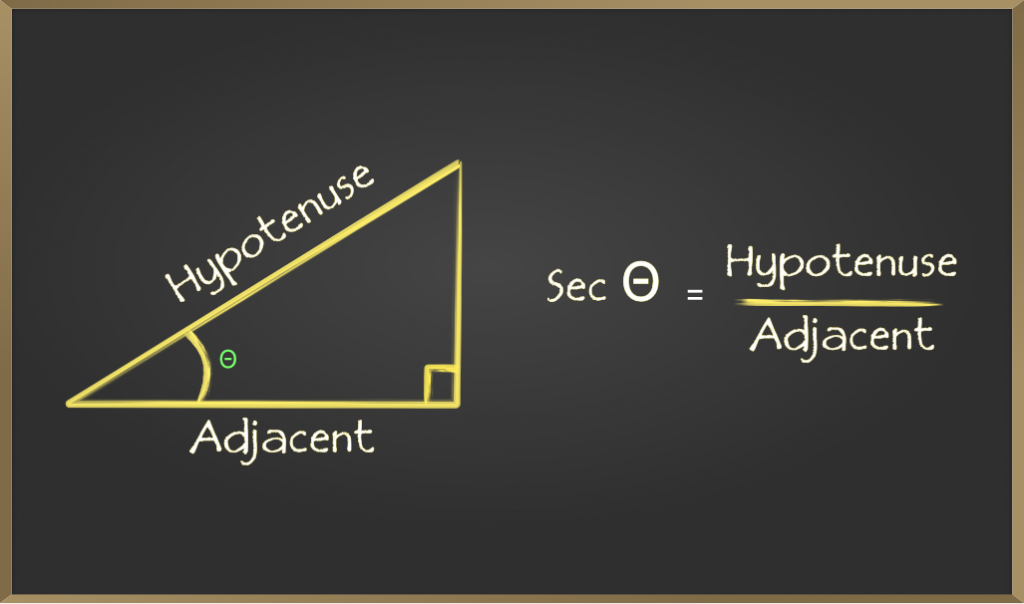## Cotangent (cot)

Cotangent of an angle is defined by the ratio of the length of sides that is adjacent to the angle and the side which is opposite to the angle. It is represented as cot θ.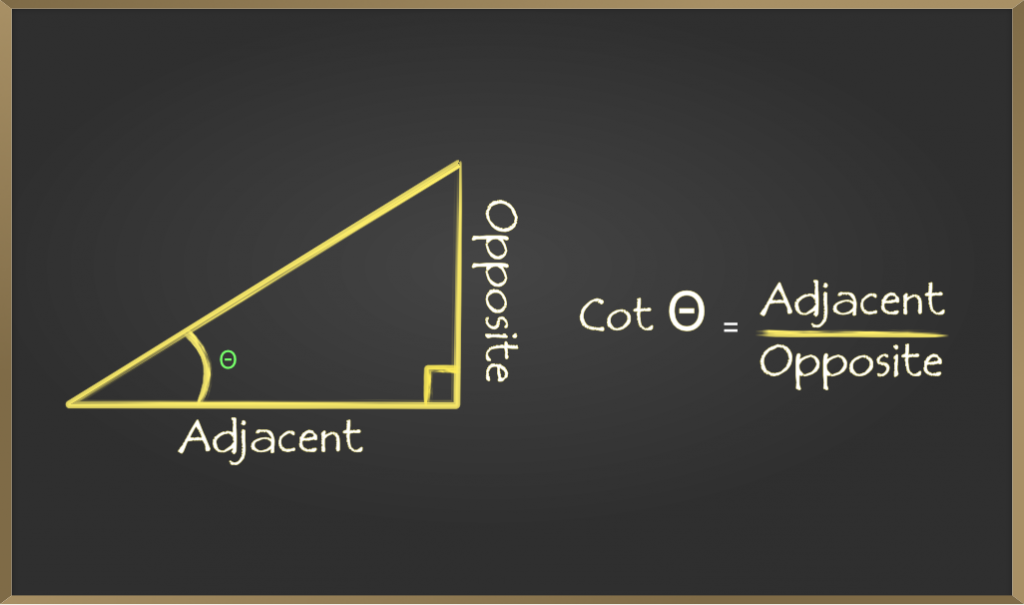## Trigonometric Ratios Table

Trigonometric ratios for any specific angle ‘θ’ is given below:

## Trigonometric Table of Some Specific Angles

Trigonometric Ratios for standard angles 0°, 30°, 45°, 60°, and 90º are required to solve trigonometric ratios. Various trigonometric identities are also required to solve trigonometric functions. Now, the value of trigonometric ratios for specific angles such as 30°, 45°, etc in the table below:

## Solving for a Side in Right Triangles with Trigonometric Ratio

This is one of the most basic and useful uses of trigonometry using the trigonometric ratios mentioned to find the length of a side of a right-angled triangle but to do, so we must already know the length of the other two sides or an angle and length of one side.

### Steps to follow if one side and one angle are known:

1. Choose a trigonometric ratio that contains the given side and the unknown side
2. Use algebra to find the unknown side

Example: In a right-angled triangle, ABC ∠B = 90° and ∠C = 30° length of side AB is 4 find the length of BC given tan 30° = 1/√3.Solution:

C = 30°
tan C = tan 30°
= 1/√3
tan C = opposite side/adjacent side
1/√3 = AB/BC
1/√3 = 4/BC
BC = 4√3

### Steps to follow if two sides are known:

1. Mark the known sides as adjacent, opposite, or hypotenuse with respect to any one of the acute angles in the triangle.
2. Decide on which trigonometric ratio can be found from the above table.
3. Find the angle (X)
4. Use a trigonometric ratio with respect to X which is a ratio of a known side and an unknown side.
5. Use algebra to find the unknown side.

Example: If two sides of a right-angled triangle are 20 and 10√3 where the side with length 20 is the hypotenuse, find the third side (without using Pythagoras theorem) given sin 30° = 1/2 and cos 30° = √3/2

Solution:

hypotenuse = 20
cos θ = adjacent side / hypotenuse
= 10√3 / 20
θ  = √3/2

## Solved Examples on Trigonometric Ratios

Example 1: Find the value of sin θ if tan θ = 4/3 and cos θ = 6/10.

Solution:

Given, tan θ = 4/3 and cos θ = 6/10
we know that,
tan θ = sin θ/cos θ
4/3 = sin θ/(6/10)
sin θ = (4/3)×(6/10)
sin θ = 8/10

Example 2: In a right-angled triangle PQR, right-angled at Q, the hypotenuse is PR = 13 cm, the base is QR = 5 cm and the perpendicular is PQ = 12 cm, and if ∠PQR = θ, then find sin θ, cos θ, and tan θ.

Solution:

Given, In ∆PQR,
Hypotenuse, PR = 13 cm
Base, QR = 5 cm
Perpendicular, PQ = 12 cm
now,
sin θ = Perpendicular/Hypotenuse = PQ/PR = 12/13
cos θ = Base/Hypotenuse = QR/PR = 5/13
tan θ = Perpendicular/Base = PQ/QR = 12/5

Example 3: Find the value of cos θ if tan θ = √3 and sin θ = √3/2.

Solution:

Given, tan θ = √3 and sin θ = √3/2
we know that,
tan θ = sin θ/cos θ
√3 = (√3/2)/cos θ
cos θ = (√3/2)/√3
cos θ = 1/2

Example 4: Find the value of sec2 θ if tan2 θ = 1

Solution:

Given, tan2 θ = 1…(1)

we know that, sec2 θ – tan2 θ = 1…(2)

By eq (1),

sec2 θ – 1 = 1
sec2 θ  = 1 + 1

than,

sec2 θ  = 2

## FAQs on Trigonometric Ratios

Question 1: What are the various real-life applications of Trigonometric Ratios?

Various real life applications of trigonometric ratios are:

• sin and cos functions are used to represent various waves and harmonic functions.
• Trigonometric Ratios are used in the study of architectural engeering, civil engeering, astronomical stuies, etc.
• Trigonometric ratios are used in getting ideaof directions and creating maps.

Question 2: Write the three main trigonometric ratios.

The three basic trigonometric ratios are sine (sin), cosine (cos), and tangent (tan)

Question 3: What are all the six trigonometric ratios?

All Six 6 trigonometric ratios are sine (sin), cosine (cos), tangent (tan), cotangent (cot), cosecant (cosec), and secant (sec).

Question 4: Write the trigonometric ratios of complementary angles.